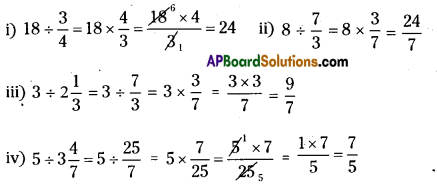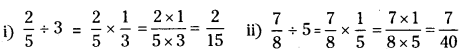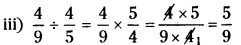# HSSlive: Plus One & Plus Two Notes & Solutions for Kerala State Board

## AP Board Class 7 Maths Chapter 2 Fractions Decimals and Rational Numbers Ex 4 Textbook Solutions PDF: Download Andhra Pradesh Board STD 7th Maths Chapter 2 Fractions Decimals and Rational Numbers Ex 4 Book AnswersAP Board Class 7 Maths Chapter 2 Fractions Decimals and Rational Numbers Ex 4 Textbook Solutions PDF: Download Andhra Pradesh Board STD 7th Maths Chapter 2 Fractions Decimals and Rational Numbers Ex 4 Book Answers

## Andhra Pradesh State Board Class 7th Maths Chapter 2 Fractions Decimals and Rational Numbers Ex 4 Books Solutions

 Board AP Board Materials Textbook Solutions/Guide Format DOC/PDF Class 7th Subject Maths Chapters Maths Chapter 2 Fractions Decimals and Rational Numbers Ex 4 Provider Hsslive

## How to download Andhra Pradesh Board Class 7th Maths Chapter 2 Fractions Decimals and Rational Numbers Ex 4 Textbook Solutions Answers PDF Online?

2. Click on the Andhra Pradesh Board Class 7th Maths Chapter 2 Fractions Decimals and Rational Numbers Ex 4 Answers.
3. Look for your Andhra Pradesh Board STD 7th Maths Chapter 2 Fractions Decimals and Rational Numbers Ex 4 Textbooks PDF.
4. Now download or read the Andhra Pradesh Board Class 7th Maths Chapter 2 Fractions Decimals and Rational Numbers Ex 4 Textbook Solutions for PDF Free.

## AP Board Class 7th Maths Chapter 2 Fractions Decimals and Rational Numbers Ex 4 Textbooks Solutions with Answer PDF Download

Find below the list of all AP Board Class 7th Maths Chapter 2 Fractions Decimals and Rational Numbers Ex 4 Textbook Solutions for PDF’s for you to download and prepare for the upcoming exams:

Question 1.
Find the reciprocal of each of the following fractions.
(i) 58
(ii) 87
(iii) 137
(iv) 34
Solution:
i) Reciprocal of 58 = 85
ii) Reciprocal of 87 = 78
iii) Reciprocal of 137 = 713
iv) Reciprocal of 34 = 43

Question 2.
Find
(i) 18 ÷ 34
(ii) 8 ÷ 73
(iii) 3 ÷ 213
(iv) 5 ÷ 347
Solution:Question 3.
Find
(i) 25 ÷ 3
(ii) 78 ÷ 5
(iii) 49 ÷ 45
Solution:Question 4.
Deepak can paint 25 of a house in one day. lfhe continues working at this rate, how many days will he take to paint the whole house?
Amount of work done by deepak in 1 day = 25 th
Total work can be done in = 1 ÷ 25 days
= 25 = 1×52=1×52=52=212 days

## Andhra Pradesh Board Class 7th Maths Chapter 2 Fractions Decimals and Rational Numbers Ex 4 Textbooks for Exam Preparations

Andhra Pradesh Board Class 7th Maths Chapter 2 Fractions Decimals and Rational Numbers Ex 4 Textbook Solutions can be of great help in your Andhra Pradesh Board Class 7th Maths Chapter 2 Fractions Decimals and Rational Numbers Ex 4 exam preparation. The AP Board STD 7th Maths Chapter 2 Fractions Decimals and Rational Numbers Ex 4 Textbooks study material, used with the English medium textbooks, can help you complete the entire Class 7th Maths Chapter 2 Fractions Decimals and Rational Numbers Ex 4 Books State Board syllabus with maximum efficiency.

## FAQs Regarding Andhra Pradesh Board Class 7th Maths Chapter 2 Fractions Decimals and Rational Numbers Ex 4 Textbook Solutions

#### Can we get a Andhra Pradesh State Board Book PDF for all Classes?

Yes you can get Andhra Pradesh Board Text Book PDF for all classes using the links provided in the above article.

## Important Terms

Andhra Pradesh Board Class 7th Maths Chapter 2 Fractions Decimals and Rational Numbers Ex 4, AP Board Class 7th Maths Chapter 2 Fractions Decimals and Rational Numbers Ex 4 Textbooks, Andhra Pradesh State Board Class 7th Maths Chapter 2 Fractions Decimals and Rational Numbers Ex 4, Andhra Pradesh State Board Class 7th Maths Chapter 2 Fractions Decimals and Rational Numbers Ex 4 Textbook solutions, AP Board Class 7th Maths Chapter 2 Fractions Decimals and Rational Numbers Ex 4 Textbooks Solutions, Andhra Pradesh Board STD 7th Maths Chapter 2 Fractions Decimals and Rational Numbers Ex 4, AP Board STD 7th Maths Chapter 2 Fractions Decimals and Rational Numbers Ex 4 Textbooks, Andhra Pradesh State Board STD 7th Maths Chapter 2 Fractions Decimals and Rational Numbers Ex 4, Andhra Pradesh State Board STD 7th Maths Chapter 2 Fractions Decimals and Rational Numbers Ex 4 Textbook solutions, AP Board STD 7th Maths Chapter 2 Fractions Decimals and Rational Numbers Ex 4 Textbooks Solutions,
Share: